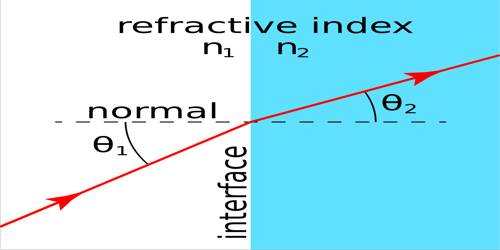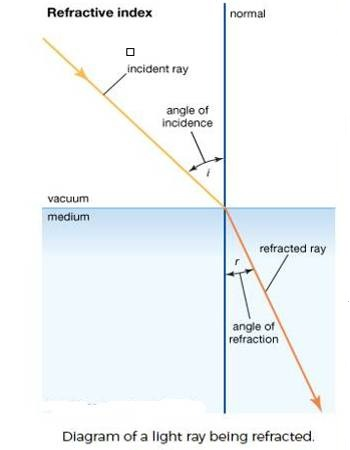# Refractive Index

Refractive index is the ratio of the velocity of light in a vacuum to its velocity in a specified medium. It is the ratio of the velocity of light in a vacuum to its velocity in a specified medium. The refractive index of liquids depends on the density but is independent of temperature. Based on purely theoretical consideration of the electromagnetic and wave theories of light H.A. Lorenz and L. Lorenz deduced the expression

r = [(n2 – 1) / (n2 + 1)] (1/d)

for the specific refraction, r, where n is the refractive index and d is the density of the liquid. They defined molar refraction, Mr, as equal to the specific refraction multiplied by molecular mass, mathematically it is given by

Mr = [(n2 – 1) / (n2 + 1)] (M/d)Refractive Index is the ratio of the speed of radiation (such as light) in one medium (such as air, glass, or a vacuum) to that in another medium. The value of the molar refraction calculated according to the expression of Lorenz and Lorenz was found to be independent of temperature and pressure but dependent on the wave-length of light used to treasure the refractive index. Refractive index is usually measured with a refractometer, the commonly used ones being Abbe refractometer and the Pulfrich’s refractometer. For the description of the instrument and the method of measurement, a book on practical physical chemistry should be consulted. For comparison purposes refractive index is measured with light of definite wave-length, usually the sodium-D line. The concept of refractive index applies within the full electromagnetic spectrum, from X-rays to radio waves. It can also be applied to wave phenomena such as sound.